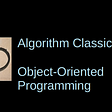# Step 1: Understanding the problem

`segments :: [Int]segments =    [2, 1, 1, 1, 2, 1, 2, 1, 1, 1, 1, 2, 1, 2, 1, 1, 1, 1, 1, 2]`
`type Pos = (Int, Int, Int)data Dir = F | B | L | R | U | D deriving (Show)move :: Dir -> Pos -> Posmove F (i, j, k) = (i + 1, j, k)move B (i, j, k) = (i - 1, j, k)move L (i, j, k) = (i, j - 1, k)move R (i, j, k) = (i, j + 1, k)move U (i, j, k) = (i, j, k + 1)move D (i, j, k) = (i, j, k - 1)`

# Step 2: Recursive tree search

`turns :: Dir -> [Dir]turns F = [L, R, U, D]turns B = [L, R, U, D]turns L = [F, B, U, D]turns R = [F, B, U, D]turns U = [F, B, L, R]turns D = [F, B, L, R]`
`data SearchState = SearchState {    currentPos :: Pos,    currentDir :: Dir,    remainingSegments :: [Int],    usedPositions :: Set Pos    }`
1. What’s the last position of a segment that I’ve decided to place?
2. What direction am I planning to move next?
3. What are the segments I haven’t placed yet?
4. Which positions already have blocks in them (since two blocks cannot land in the same position)?
`initialSearchStates :: [SearchState]initialSearchStates = [    SearchState {        currentPos = (0, j, k),        currentDir = F,        remainingSegments = segments,        usedPositions = Set.empty    }    | j <- [0..2], k <- [0..2] ]`
`goodPosition :: Set Pos -> Pos -> BoolgoodPosition used pos =    inRange ((0,0,0),(2,2,2)) pos && not (pos `Set.member` used)`
`search :: SearchState -> [[Dir]]search SearchState{ remainingSegments = [] } = [[]]search SearchState{..}  | all (goodPosition usedPositions) cover        = [ currentDir : solution          | dir' <- turns currentDir          , solution <- search SearchState{              currentPos = last covered,              currentDir = dir',              remainingSegments = segs,              usedPositions = foldr Set.insert usedPositions                                    (init cover)              }          ]  | otherwise = []  where s:segs = remainingSegments        cover = take (s + 1) (iterate (move currentDir) currentPos)`
`theAnswer :: [Dir]theAnswer = head (concatMap search initialSearchStates)`

# Step 3: Visualization

`blocks :: [Pos]blocks = follow (0, 0, 0) (zip theAnswer segments)  where follow p [] = [p]        follow p ((_, 0) : steps) = follow p steps        follow p ((d, n) : steps)             = p : follow (move d p) ((d, n - 1) : steps)`
`main :: IO ()main = activityOf initial change pictureinitial :: Intinitial = 1change :: Event -> Int -> Intchange (KeyPress "Up")   n = n + 1change (KeyPress "Down") n = n - 1change other             n = n`
1. The pictures of individual blocks must be overlapped in the right order, so that blocks nearest the user obscure the blocks further away.
2. The sides must be projected from three dimensions into two. I chose an isometric projection, so that I can be sure there are only three visible sides.
`picture :: Int -> Picturepicture n = pictures [    drawBlock p    | p <- sortBy (comparing viewSortKey) (take n blocks)    ]  where viewSortKey (i, j, k) = (i, -j, -k)drawBlock :: Pos -> PicturedrawBlock (i, j, k) = pictures [    -- The front face.    colored (light gray) \$ solidPolygon [        project p | p <- [ (x + 0.5, y + 0.5, z - 0.5),                           (x + 0.5, y - 0.5, z - 0.5),                           (x - 0.5, y - 0.5, z - 0.5),                           (x - 0.5, y + 0.5, z - 0.5) ] ],    -- The top face.    colored gray \$ solidPolygon [        project p | p <- [ (x + 0.5, y + 0.5, z + 0.5),                           (x + 0.5, y + 0.5, z - 0.5),                           (x - 0.5, y + 0.5, z - 0.5),                           (x - 0.5, y + 0.5, z + 0.5) ] ],    -- The right face.    colored (dark gray) \$ solidPolygon [        project p | p <- [ (x + 0.5, y + 0.5, z + 0.5),                           (x + 0.5, y + 0.5, z - 0.5),                           (x + 0.5, y - 0.5, z - 0.5),                           (x + 0.5, y - 0.5, z + 0.5) ] ]    ]  where x = fromIntegral j        y = fromIntegral k        z = fromIntegral iproject :: (Double, Double, Double) -> Pointproject (x, y, z) = (3 * x + (1 + sin t / 4) * z,                     3 * y + (1 + cos t / 4) * z)`

--

--

--

## More from Chris Smith

Software engineer, volunteer K-12 math and computer science teacher, author of the CodeWorld platform, amateur ring theorist, and Haskell enthusiast.

Love podcasts or audiobooks? Learn on the go with our new app.

## The History of Hello World.## Flutter And Firebase Are An Assassin Combination For The App Development## Functional Reactive Programming with Reflex and CodeWorld## 3 must-have customizations for a user-friendly Dynamics 365## A Newbie’s Guide to Bug Bashing## Part-of-Speech Tag a String, Filter to Adverbs in Go## New Update: HashiCorp Ambassador Award 2021 !## Report, Restore, Resolve, Root-cause Incidents## Chris Smith

Software engineer, volunteer K-12 math and computer science teacher, author of the CodeWorld platform, amateur ring theorist, and Haskell enthusiast.

## Rosetta Code: Object Oriented Programming Examples